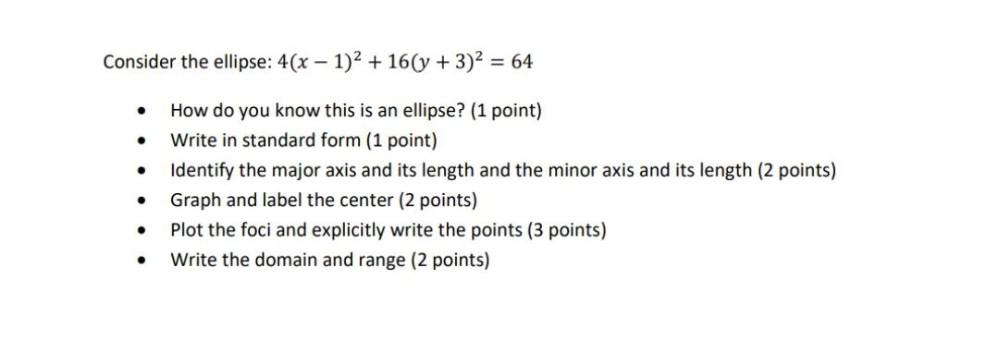Question:

# Consider the ellipse: 4(x - 1)^2 + 16(y + 3)^2 = 64 • How do you know this is an ellipse? • Write in standard form. • Identify the major axis and its length and the minor axis and its length. • GraphConsider the ellipse: 4(x - 1)^2 + 16(y + 3)^2 = 64 • How do you know this is an ellipse? • Write in standard form. • Identify the major axis and its length and the minor axis and its length. • Graph and label the center. • Plot the foci and explicitly write the points. • Write the domain and range.